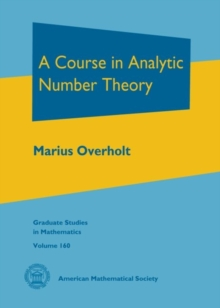# A Course in Analytic Number Theory Hardback

## Part of the Graduate Studies in Mathematics series

Hardback

• Information

#### Description

This book is an introduction to analytic number theory suitable for beginning graduate students.

It covers everything one expects in a first course in this field, such as growth of arithmetic functions, existence of primes in arithmetic progressions, and the Prime Number Theorem.

But it also covers more challenging topics that might be used in a second course, such as the Siegel-Walfisz theorem, functional equations of L-functions, and the explicit formula of von Mangoldt.

For students with an interest in Diophantine analysis, there is a chapter on the Circle Method and Waring's Problem.

Those with an interest in algebraic number theory may find the chapter on the analytic theory of number fields of interest, with proofs of the Dirichlet unit theorem, the analytic class number formula, the functional equation of the Dedekind zeta function, and the Prime Ideal Theorem. The exposition is both clear and precise, reflecting careful attention to the needs of the reader.

The text includes extensive historical notes, which occur at the ends of the chapters.

The exercises range from introductory problems and standard problems in analytic number theory to interesting original problems that will challenge the reader. The author has made an effort to provide clear explanations for the techniques of analysis used.

No background in analysis beyond rigorous calculus and a first course in complex function theory is assumed.

#### Information

• Format:Hardback
• Pages:371 pages
• Publisher:American Mathematical Society
• Publication Date:
• Category:
• ISBN:9781470417062

£77.50

£72.96

£81.95

£76.89

£90.50

£84.47

£114.00

£105.98

£81.95

£76.89